GFG App
Open AppBrowser
Continue

In Mathematics, we have learned formulas to calculate the area of various figures such as circles, squares, rectangles, spheres, etc. but apart from these figures we also come across non-linear figures or curves that do not have a direct formula to calculate the area under them. The integration method aids in determining the area of such figures. The antiderivative techniques are highly useful for locating the regions of irregular plane surfaces. In this article, we shall learn how to find the area under a curve.

## Area under the Curve

The area under a curve can be calculated with respect to different axes such as the Y- axis and X- axis. By performing a definite integral between the two locations, one may determine the area under a curve between two points. Integrate y = f(x) between the limits of a and b to determine the area under the curve y = f(x) between x = a & x = b. With the specified restrictions, integration may be used to compute this area.

### Area with Respect to X-Axis

The curve shown in the image below is represented using y = f(x). We need to calculate the area under the curve with respect to X-axis. The boundary values for the curve on the X-axis are a and b respectively. The area A under this curve with respect to X-axis is calculated between the points x = a  and x = b. Consider the following curve: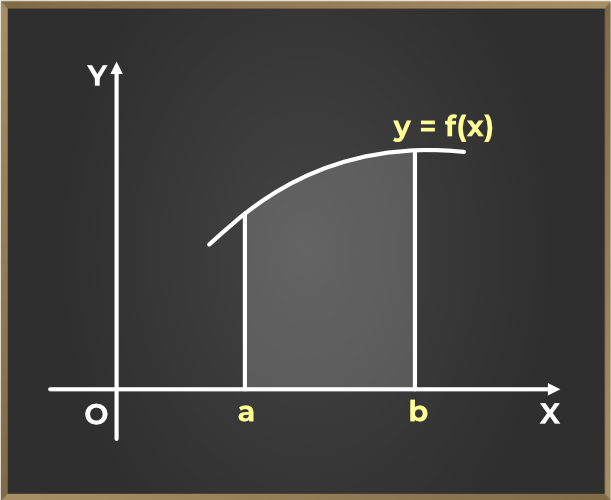### Formulaorwhere,

• A is the area under the curve
• y or f(x) is the equation of the curve
• a, b are the x-intercepts

### Area with Respect to Y-Axis

The curve shown in the image above is represented using x = f(y). We need to calculate the area under the curve with respect to Y-axis. The boundary values for the curve on the Y-axis are a and b respectively. The area A under this curve with respect to Y-axis between the points y = a and y = b. Consider the following curve: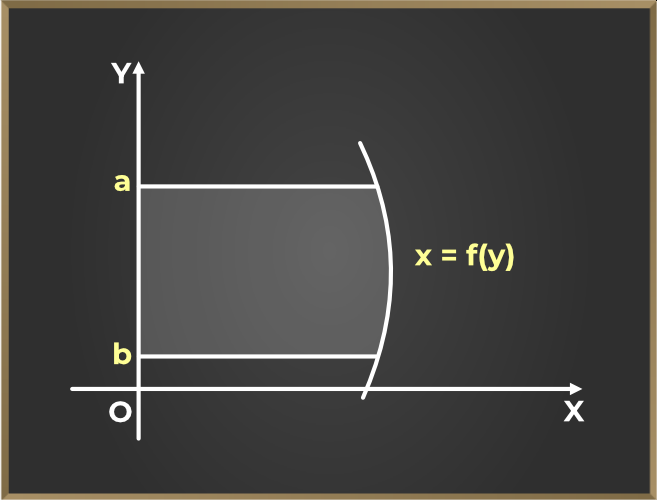### Formulaorwhere,

• A is the area under the curve
• x or f(y) is the equation of the curve
• a, b are the y-intercepts

## Solved Examples on Area Under The Curve

Example 1: Find the area under the curve y2 = 12x and the X-axis.

Solution:

The given curve equation is y2 = 12x.

This is an equation of parabola with a = 3 so, y2 = 4(3)(x)

The graph for the required area is shown below: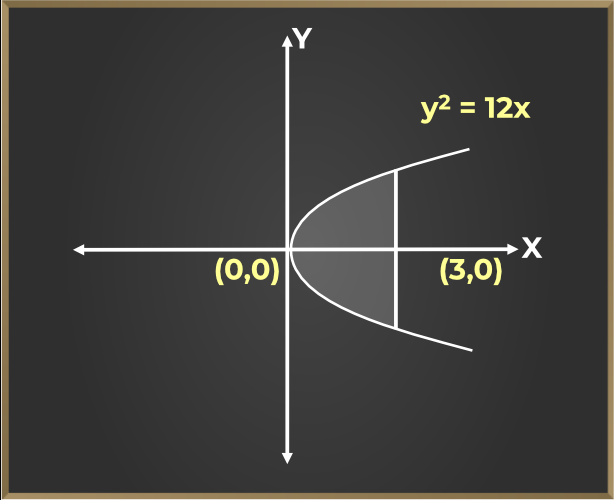The X-axis divides the above parabola into 2 equal parts. So, we can find the area in the first quadrant and then multiply it by 2 to get the required area.

So, we can find the required area as:A = 24 sq. units

Example 2: Calculate the area under the curve x = y3 – 9 between the points y = 3 and y = 4.

Solution:

Given, the equation of curve is x = y3 – 9.

The boundary points are (0, 3) and (0, 4) .

As the equation of curve is of the form x = f(y) and the points are also on the Y-axis, we will use the formula,A = 139/4 sq. units

Example 3: Calculate the area under the curve y = x2 – 7 between the points x = 5 and x = 10.

Solution:

Given, the curve is y = x2−7 and the boundary points areandThus, the area under the curve is given by:A = 770/3 sq. units

Example 4: Find the area enclosed by the parabola y2 = 4ax and the line x = a in the first quadrant.

Solution:

The curve and the line given can be drawn as follows: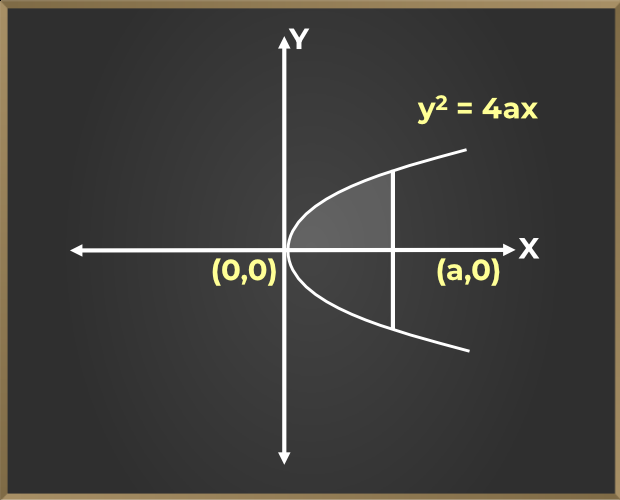Now, the equation of curve is y2 = 4ax.

The boundary points come out to be (0,0) and (a,0).

So the area with respect to X-axis can be calculated as:Example 5: Find the area covered by the circle x2 + y2 = 25 in the first quadrant.

Solution:

Given, x2 + y2 = 25.

The curve can be drawn as: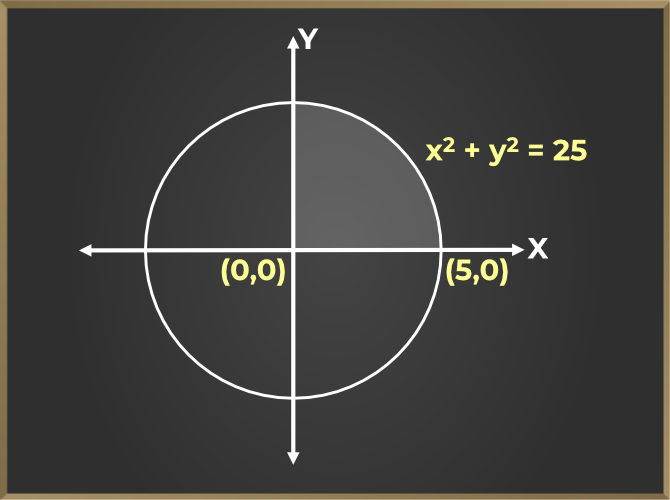The required area has been shaded in the above figure. From the equation we can see that radius of the circle is 5 units.

As, x2 + y2 = 25To find the area, we shall use:A = 25 π/4 sq. units

## FAQs on Area Under The Curve

Question 1: Explain the meaning of the area under the curve.

Solution:

The region enclosed by the curve, the axis, and the boundary points is referred to as the area under the curve. Using the coordinate axes and the integration formula, the area under the curve has been determined as a two-dimensional area.

Question 2: Explain the three methods to find the area under the curve.

Solution:

Three methods to find the area under the curve are:

• The first method is to break up the space into multiple tiny rectangles of the area under the curve. The areas are then summed to get the overall area.
• The second technique involves cutting the space into a few rectangles, which are then joined together to create the desired area.
• Utilizing integration to locate the region is the third approach.

Question 3: Can the area under the curve be negative? If yes, explain.

Solution:

If the curve is below the axis or lies in the coordinate axis’s negative quadrants, the area under the curve is negative. In this case as well, the area under the curve is computed using the conventional approach, and the solution is then modulated. Even in cases when the answer is negative, just the area’s value is taken into account, not the answer’s negative sign.

Question 4: How is the area under the curve approximated?

Solution:

By segmenting the region into tiny rectangles, the area under the curve may be roughly estimated. And by adding the areas of these rectangles, one may get the area under the curve. A collection of a few large rectangles may be drawn, and their areas can then be added to determine the approximate area under the curve. Additionally, with the use of definite integrals, we can easily determine the precise area under the curve.

### Related Resources

My Personal Notes arrow_drop_up